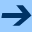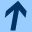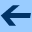On the formal second cocycle equation for iteration groups of type II

## On the formal second cocycle equation for iteration groups of type II

Jointly written with LUDWIG REICH.

Journal of Difference Equations and Applications 21 Issue 7, (2015). DOI 10.1080/10236198.2015.1040783

Abstract. Let x be an indeterminate over ℂ. We investigate solutions

 β(s,x)=∑n≥ 0βn(s)xn,..
βn: ℂ→ ℂ, n≥ 0, of the second cocycle equation
 β(s+t,x)= β(s,x)α(t,F (s,x)) +β(t,F (s,x)),         s,t∈ ℂ                        (Co2)..
in C[[x]], the ring of formal power series over ℂ, where (F(s,x))s∈ ℂ is an iteration group of type II, i.e. it is a solution of the translation equation
 F(s+t,x)=F(s,F(t,x)),        s,t∈ ℂ,                         (T)..
of the form F(s,x)≡ x+ck(s)xkmodxk+1, where k≥ 2 and ck ≠ 0 is necessarily an additive function. Moreover, (α(s,x))s∈ ℂ is a solution of the first cocycle equation
 α(s+t,x)= α(s,x)α(t,F (s,x)),        s,t∈ ℂ.                         (Co1)..
Using the method of "formal functional equations" applied already for the study of F and α in previous manuscripts we obtain a formal version of the second cocycle equation in the ring (ℂ[S,U,σ])[[x]]. We solve this equation in a completely algebraic way, by deriving formal differential equations. This way it is possible to get the structure of the coefficients in great detail which are now polynomials. We prove the universal character of these polynomials depending on certain parameters, the coefficients of the generator K of a formal first cocycle, and the coefficients of three generators N1,N2,N3 of a formal second cocycle for iteration groups of type II.
harald.fripertinger "at" uni-graz.at, March 4, 2019